# Loss function

(diff) ← Older revision | Latest revision (diff) | Newer revision → (diff)
In a problem of statistical decision making, a non-negative function indicating the loss (cost) to an experimenter given a particular state of the world and a particular decision. Letbe a random variable taking values in a sample space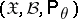,, and let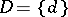be the space of all possible decisions that can be taken on the basis of an observed. In the theory of statistical decision functions, any non-negative functiondefined on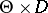is called a loss function. The value of a loss functionat an arbitrary point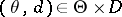is interpreted as the cost incurred by taking a decision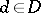, when the true parameter is,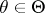.